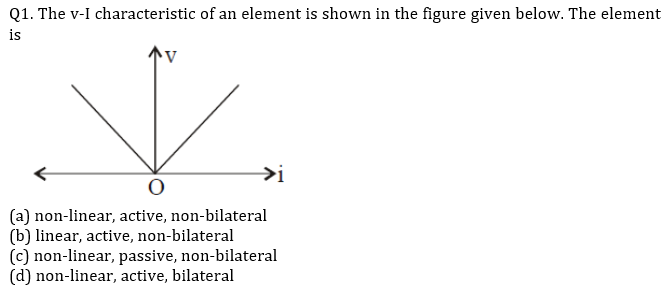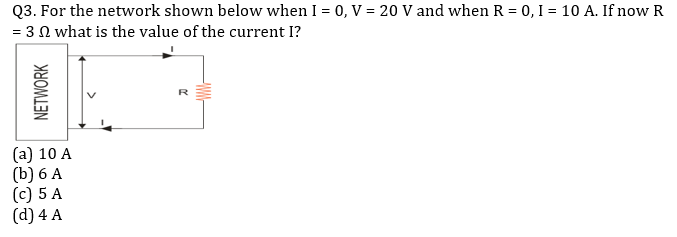Engineering Jobs   »   ELECTRICAL GATE QUIZ,

# GATE’22 EE: Daily Practices Quiz 16-July-2021Q2. For the idealized half wave rectified system the average and rms value of the voltage is
(a) V_m/π,V_m/2
(b) V_m/π,V_m/√2
(c) 〖2V〗_m/π,V_m/2
(d) 〖2V〗_m/π,V_m/√2Q4. An open loop system represented by the transfer function G(s)=((s-1))/((s+2)(s+3)) is
(a) Stable and of minimum phase type
(b) Stable and of non-minimum phase type
(c) Unstable and of minimum phase type
(d) Unstable and of non-minimum phase type

Q5. A 220 V dc shunt motor takes a total current of 80 A and runs at 800 rpm. Resistance
Of shunt field is 50 Ω and that of armature 0.1 Ω. The iron and friction losses amount to
1600 W. What is the driving power of the motor?
(a) 16050 W
(b) 14500 W
(c) 12600 W
(d) 18500 W

SOLUTIONS

S1. Ans.(a)
Sol. For bilateral element v and i both are negative in reverse direction. The given v-i characteristic represent charging and discharging of a voltage source which is an active element.
Bilateral Elements are the elements that allow the current in both directions and offer the same impedance in either direction of current flow.
Non-Linear Elements are those that do not show a linear relation between voltage and current.
V-I characteristics of bi-lateral elements are symmetric on 1st & 3rd or 2nd & 4th quadrant.
If at any point of V-I curve, if the slope is negative then element has active behaviour.

S2. Ans.(a)
Sol. for half wave rectifier:
V_avg=V_m/π
V_rms=V_m/2

S3. Ans.(d)
Sol. From the given data, the resistance of the network is,R_th=V_oc/I_sc =20/10=2 Ω
Total resistance= 2+3=5 Ω
So, current(I)=20/5=4 A

S4. Ans.(b)
Sol. G(s)=((s-1))/((s+2)(s+3))
For given transfer function, one zero is in right half of s- plane, so it is non-minimum phase
type. For given system, both poles on left side of s-plane, hence it is a stable system.

S5. Ans.(a)
Sol. I_sh=220/50=4.4 A;I_a=80-4.4=75.6 A
E_b=V-I_a R_a=220-75.6×0.1=212.44 V
∴ Driving power = E_b I_a=212.44×75.6 ⋍16050 W

Sharing is caring!

×

Thank You, Your details have been submitted we will get back to you.Join India's largest learning destination

What You Will get ?

•Job Alerts
•Daily Quizzes
•Subject-Wise Quizzes
•Current Affairs
•Previous year question papers
•Doubt Solving session

ORJoin India's largest learning destination

What You Will get ?

•Job Alerts
•Daily Quizzes
•Subject-Wise Quizzes
•Current Affairs
•Previous year question papers
•Doubt Solving session

ORJoin India's largest learning destination

What You Will get ?

•Job Alerts
•Daily Quizzes
•Subject-Wise Quizzes
•Current Affairs
•Previous year question papers
•Doubt Solving session

Enter the email address associated with your account, and we'll email you an OTP to verify it's you.Join India's largest learning destination

What You Will get ?

•Job Alerts
•Daily Quizzes
•Subject-Wise Quizzes
•Current Affairs
•Previous year question papers
•Doubt Solving session

Enter OTP

Please enter the OTP sent to
/6

Did not recive OTP?

Resend in 60sJoin India's largest learning destination

What You Will get ?

•Job Alerts
•Daily Quizzes
•Subject-Wise Quizzes
•Current Affairs
•Previous year question papers
•Doubt Solving sessionJoin India's largest learning destination

What You Will get ?

•Job Alerts
•Daily Quizzes
•Subject-Wise Quizzes
•Current Affairs
•Previous year question papers
•Doubt Solving session

Almost there

+91Join India's largest learning destination

What You Will get ?

•Job Alerts
•Daily Quizzes
•Subject-Wise Quizzes
•Current Affairs
•Previous year question papers
•Doubt Solving session

Enter OTP

Please enter the OTP sent to Edit Number

Did not recive OTP?

Resend 60

By skipping this step you will not recieve any free content avalaible on adda247, also you will miss onto notification and job alerts

Are you sure you want to skip this step?

By skipping this step you will not recieve any free content avalaible on adda247, also you will miss onto notification and job alerts

Are you sure you want to skip this step?